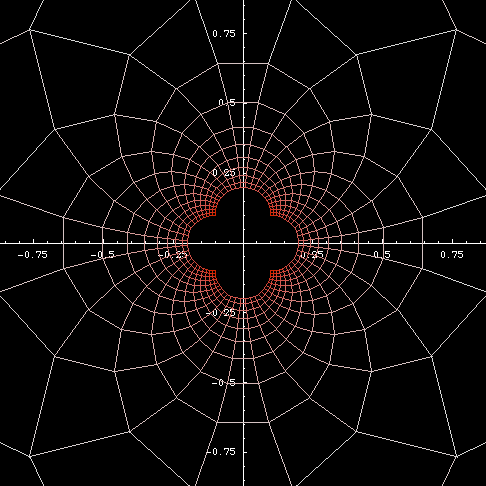# The Geometric Significance of Complex Conjugate

By Xah Lee. Date: . Last updated: .

This essay is some personal notes in math study. It explains the geometric significance of complex conjugate.Geometric inversion of a rectangular grid. Geometric Inversion is 1/Conjugate[z] [see Geometric Inversion]

In the past few years, i've been reading math haphazardly, and in the process, i have learned a few things that has puzzled me for a long time. Few weeks ago, i discovered another one, namely, the importance of conjugate operation for complex numbers. I realized this when i was trying to do inverse rotation with complex numbers.

The conjugate of a complex number {x,y} is defined as {x,-y}. Here's the definition using different notations:

`Conjugate[{a,b}] := {a, -b}`
`Conjugate[a+b*ⅈ] := a - b*ⅈ`

Conjugate is often written with a bar over. That is, conjugate of a complex number z is written as z̅.

In complex numbers texts, the conjugate is simply just defined in the outset, without qualification and explanations whatsoever. This is a general problem with modern math textbooks, where the origin of a need of a important definition is almost never mentioned, instead, axiomatic, opaque, condensed, “clean” definitions are given in the outset. Then, important theorems are given, with proofs using these opaque definitions often becomes very simple but leaves the reader unsatisfied, as if some magical and remote computation seems to resolve some question.

When studying complex analysis, one often puzzles, why you introduce to me this magical definition. Why isn't any other arbitrary definition given? I would think, these magical definitions is used as a trick to deceive me, so that later, proofs of difficult theorem are cheated because these superficial definitions. (similar things can be said for much math text in other fields, such as matrix multiplication, algebraic curves, group theory, exponential function with complex number as power, etc)

## Conjugate = Reflection

The essence of the conjugate operation for complex numbers, from the geometric point of view, is that it is a reflection in the plane (alone the x-axis).

In algebraic perspective, the essence of the conjugate, is that it expresses the multiplicative inverse of a given complex number.

## Why is Reflection Important for the Complex Plane?

Why is reflection itself important? To answer this, we need to trace back the geometric significance of complex numbers.

The gist of complex numbers itself, is that it specifies rotation in terms of 2 points. In other words, it adds to the concept of 2D vectors a “special” multiplication, such that rotations of the plane around the origin can be expressed in terms of the component of 2 vectors. This quality, makes it possible to have operations of addition and multiplication of 2D “vectors”, is the essence and constitute the entire foundation of complex number structure. Of course, complex numbers from the algebraic point of view, would claim that the defining quality is the square root of -1 being some magical new entity “i” and thus forming a “closure” and algebraic structure of complex numbers. (which perspective is more important depends on whether taking the algebraic point of view or geometric point of view. They both, of course, amount to the same thing.)

Now, if you take the geometric point of view, and accept that the essence of complex numbers is the rotation around origin defined by its multiplication, then, the importance of conjugate operation becomes more obvious. Consider a 2D vector, we interpret vector addition as translation, which is one of the important concept of rigid motion (aka isometry). Now, rotation is another important rigid motion, and now it has multiplication operation defined. The other important rigid motion, is reflection, and this is then the complex conjugate. So now, all the rigid motions: translation, rotation, reflection, all have a convenient operation defined in terms of vector's components.

Here's another example to see why reflection is important. Often, we want to do the reverse of a rigid motion. For example, if a vector is translated by a vector {a,b}, the reverse rigid motion is translating it by {-a,-b}. They corresponds to vector addition and its inverse: vector subtraction. Now, to rotate a vector {x,y} around origin by a angle formed by the unit vector {a,b}, we do complex multiplication {x,y}⊗{a,b}. Now, how to reverse this rotation? Of course, by multiplying with a vector that has the negative angle of {a,b}. The vector with negative angle of {a,b} is {a,-b}, its conjugate!

In studying math in my pre-calculus and calculus days in about 1991, i've been wanting to know, how to rotate a point. That is, given 2 points {a,b}, what's the new coordinate if we want to rotate it by α angles around a point {c,d}. This is actually quite simple, but never really explained in “pre-calculus” courses or texts. It took me quite a few years to realize it, in about 1992 when i learned linear algebra from a great teacher Gene Seelbach at Foothill College. As we know, to rotate a point {a,b} around origin with angle α, the new coordinate is {x Cos[α] - y Sin[α], x Sin[α] + Cos[α] y}, which typically is treated as a multiplication by a matrix. Now, after about 10 years, i understood the clear connection and equivalence to complex numbers multiplication. (For detail, see Coordinate Transformation)

Some other things i've learned in math in recent years include:

Yesterday, i understood how and why 5 points defines a conic section, when reading Elementary Geometry of Algebraic Curves Buy at amazon by C G Gibson. (by supplying n pairs of solutions to a system of polynomial of degree 2 in its general form. When n is 5, the equation's coefficients are uniquely determined, up to a scalar multiplication.)

If you have a question, put \$5 at patreon and message me.